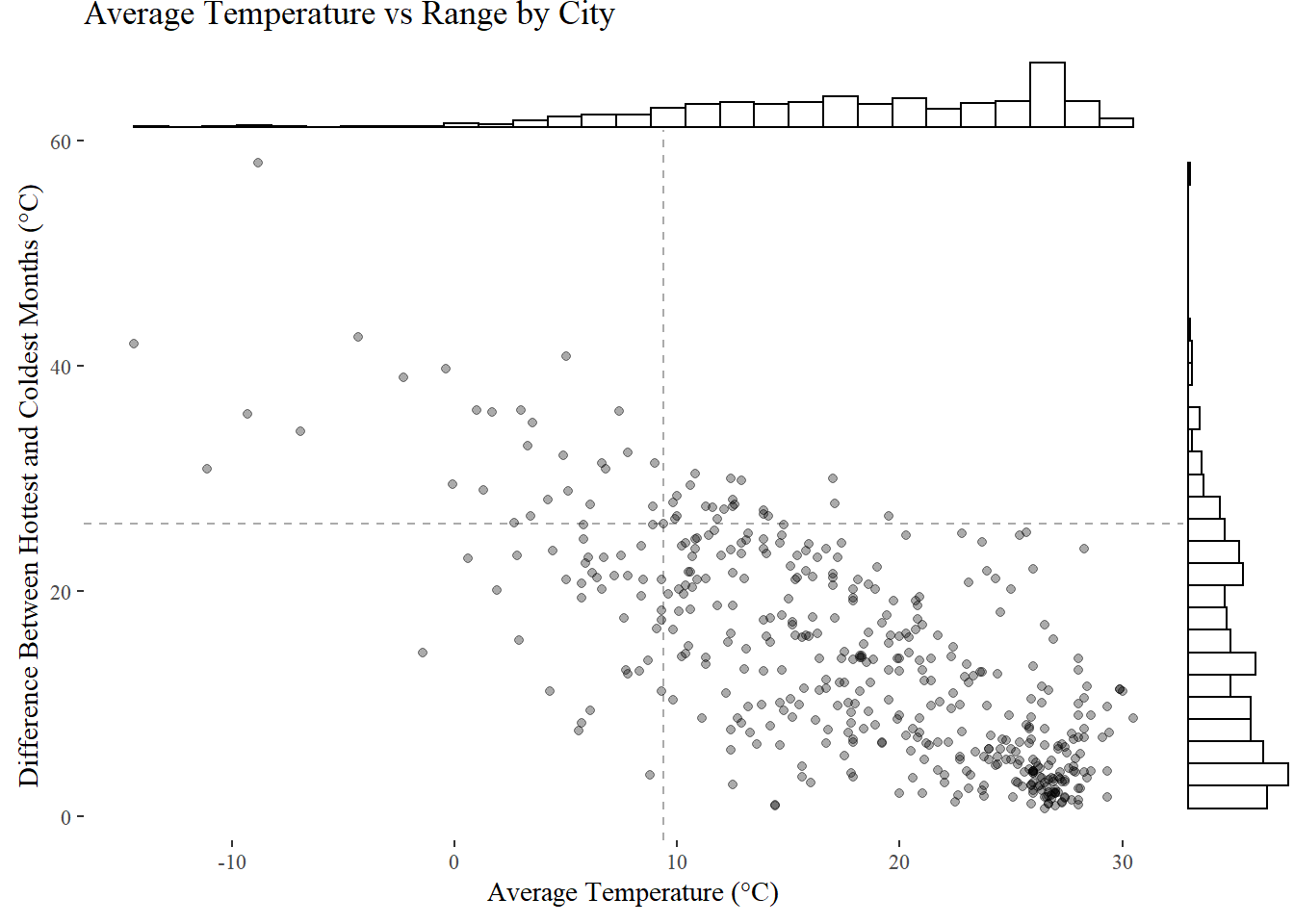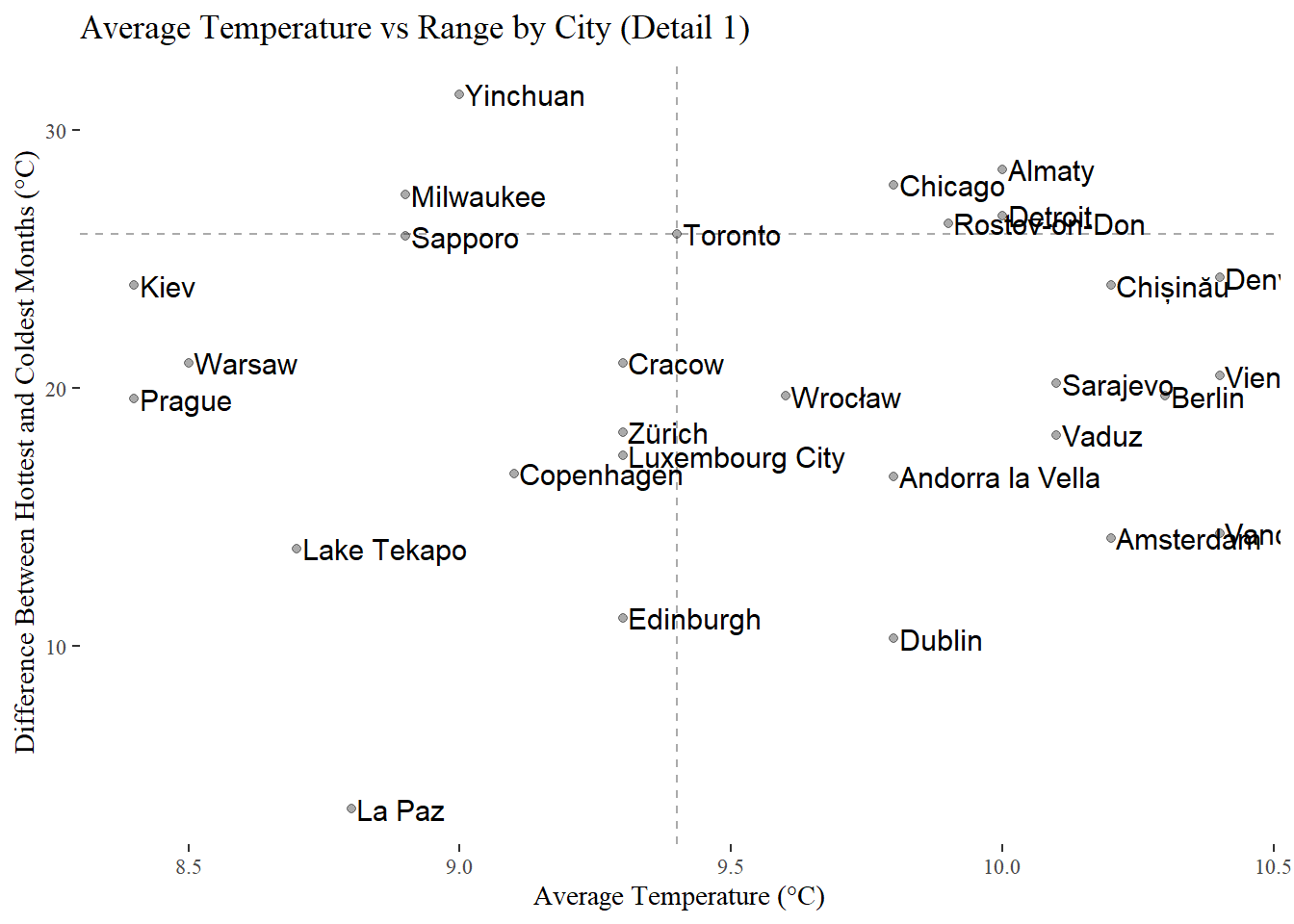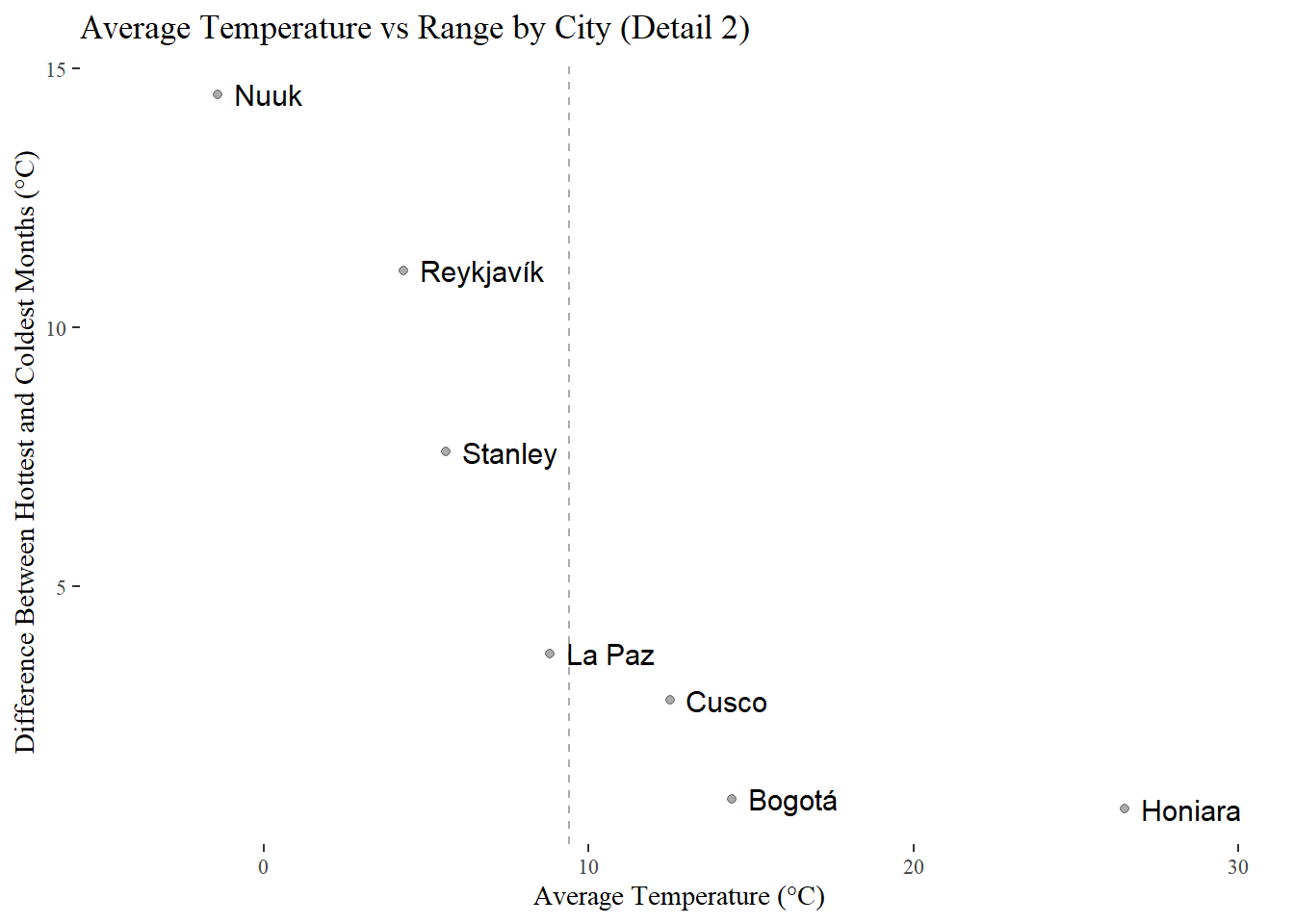## Blog Post## Introduction

I live near Toronto. It’s springtime, and currently about 30 °C. In my opinion, Toronto is too hot in the summer and too cold in the winter. I’d like to know which cities have the least deviation from a tolerable average temperature.

## Data

First, I created a CSV file comprising all the information in the Wikpedia article List of cities by average temperature.

``city_temps ``
 Algeria Algiers 11.2 11.9 12.8 14.7 17.7 21.3 24.6 25.2 23.2 19.4 15.2 12.1 17.4 Algeria Tamanrasset 12.8 15 18.1 22.2 26.1 28.9 28.7 28.2 26.5 22.4 17.3 13.9 21.7 Algeria Reggane 16 18.2 23.1 27.9 32.2 36.4 39.8 38.4 35.5 29.2 22 17.8 28.3 Angola Luanda 26.7 28.5 28.6 28.2 27 23.9 22.1 22.1 23.5 25.2 26.7 26.9 25.8 Benin Cotonou 27.3 28.5 28.9 28.6 27.8 26.5 25.8 25.6 26 26.7 27.6 27.3 27.2 Benin Parakou 26.5 28.7 29.6 29 27.5 26.1 25.1 24.7 25 26.1 26.6 26.1 26.8

Each row corresponds to a distinct city. There are two text columns containing each city’s name and country, twelve numeric columns indicating the “averages of the daily highs and lows” for each month, and one additional numeric column containing the same figure for the entire year. The units are degrees Celsius. 455 cities are included in the data.

“Average of the daily highs and lows” seems a bit ambiguous, but no matter. The article also points out that “the actual daytime temperature in a given month will be 3 to 10 °C higher than the temperature listed here, depending on how large the difference between daily highs and lows is.” It would be better to have detailed time series for each city, but we’ll press on with the data we have.

We’ll define the “deviation” mentioned above as the difference between the value recorded for the coldest and hottest months, and the “average” as the value recorded for the whole year overall.

``````city_temps
rename_with(tolower) |>
rowwise() |>
mutate(
avg=year,
range=max(c_across(jan:dec)) - min(c_across(jan:dec))
) |>
select(city, avg, range)

 Algiers 17.4 14 Tamanrasset 21.7 16.1 Reggane 28.3 23.8 Luanda 25.8 6.5 Cotonou 27.2 3.3 Parakou 26.8 4.9

## Summary Statistics

Now we can investigate the distribution of each of our two variables.

Here’s the default summary:

``````city_temps |>
select(avg, range) |>
summary()``````
``````##       avg             range
##  Min.   :-14.40   Min.   : 0.70
##  1st Qu.: 12.45   1st Qu.: 5.65
##  Median : 18.60   Median :12.10
##  Mean   : 18.00   Mean   :13.75
##  3rd Qu.: 25.65   3rd Qu.:21.00
##  Max.   : 30.50   Max.   :58.10``````

Which cities correspond to the extremes for each variable?

``````city_temps |>
filter(
avg   %in% (city_temps |> pull(avg)   |> range()) ||
range %in% (city_temps |> pull(range) |> range())
) |>
arrange(avg)``````
 Gjoa Haven -14.4 42 Yakutsk -8.8 58.1 Honiara 26.5 0.7 Assab 30.5 8.7

Let’s see the values for Toronto as a baseline, and save them for later:

``````city_temps |>
filter(city=="Toronto")``````
``````toronto_avg
filter(city=="Toronto") |>
pull(avg)

toronto_range
filter(city=="Toronto") |>
pull(range)``````

By global standards, Toronto is cool on average, but in keeping with my subjective perception, the deviation from that average over the year is quite large.

## Plots

Let’s look at a scatter plot with marginal histograms:

``````plot
ggplot(aes(x=avg, y=range)) +
geom_point(alpha=0.33) +
geom_vline(
xintercept=toronto_avg,
linetype="dashed",
alpha=0.33
) +
geom_hline(
yintercept=toronto_range,
linetype="dashed",
alpha=0.33
) +
labs(
title="Average Temperature vs Range by City",
x="Average Temperature (°C)",
y="Difference Between Hottest and Coldest Months (°C)"
) +
theme_tufte()

plot
ggMarginal(type="histogram", fill="transparent", size=10)

plot``````Here Toronto is indicated by the dashed lines.

We can see there’s a negative association between a city’s average temperature and the range of temperatures experienced there. In particular, there’s a big cluster of very hot cities which have little difference between their hottest and coldest months.

Ten tropical cities fall into both the hottest decile and the least varying decile:

``````city_temps |>
filter(
range  quantile(city_temps\$avg,   0.9)
) |>
pull(city) |>
pander()``````

Lodwar, Palembang, Pontianak, Kuala Lumpur, Malé, Lanka Colombo, Oranjestad, Willemstad, Panama City and Barranquilla

## Zooming In

The area of this plot I’m most interested in is the vertical slice around Toronto. Let’s see the same plot, including only the cities within one degree of Toronto’s average temperature. We’ll exclude the marginal histograms but add labels to the cities.

``````city_temps |>
filter(abs(avg - toronto_avg)
ggplot(aes(x=avg, y=range, label=city)) +
geom_point(alpha=0.33) +
geom_text(size=4, nudge_x=0.01, hjust="left") +
geom_vline(
xintercept=toronto_avg,
linetype="dashed",
alpha=0.33
) +
geom_hline(
yintercept=toronto_range,
linetype="dashed",
alpha=0.33
) +
labs(
title="Average Temperature vs Range by City (Detail 1)",
x="Average Temperature (°C)",
y="Difference Between Hottest and Coldest Months (°C)"
) +
theme_tufte()``````So it seems that La Paz, Edinburgh, or Dublin might be good options.

But which cities are the best? These would be the ones with the smallest range for a given maximum average. Let’s find them.

## Finding the Cities with the Nicest Weather

We want to know, for each maximum average temperature, the city that has the minimum range of temperatures. These are the cities that form the “bottom-left edge” of our first plot.

Nine cities fit this criterion:

``````city_temps |>
arrange(avg) |>
cbind(city_temps |> arrange(avg) |> pull(range) |> cummin()) |>
rename(running_min=4) |>
filter(range==running_min) |>
select(-running_min) |>
pull(city) |>
pander()``````

Gjoa Haven, Dikson, Nuuk, Reykjavík, Stanley, La Paz, Cusco, Bogotá and Honiara

Of these, the first two have temperatures which are more variable than Toronto, so we can remove them from consideration.

Let’s plot the final seven candidates:

``````city_temps |>
arrange(avg) |>
cbind(city_temps |> arrange(avg) |> pull(range) |> cummin()) |>
rename(running_min=4) |>
filter(range==running_min) |>
select(-running_min) |>
filter(range
ggplot(aes(x=avg, y=range, label=city)) +
geom_point(alpha=0.33) +
geom_text(size=4, nudge_x=0.5, hjust="left") +
geom_vline(
xintercept=toronto_avg,
linetype="dashed",
alpha=0.33
) +
scale_x_continuous(expand=expansion(mult=0.15)) +
labs(
title="Average Temperature vs Range by City (Detail 2)",
x="Average Temperature (°C)",
y="Difference Between Hottest and Coldest Months (°C)"
) +
theme_tufte()``````Again we see that La Paz has a similar overall average temperature to Toronto, but much less annual variability. Cusco and Bogotá are warmer but even less variable.

Reykjavík and Stanley are colder than Toronto, and while they represent a smaller decrease in variability compared to La Paz, Cusco, and Bogotá, they have the benefit (for me) of being 98%+ English-speaking.

Nuuk and Honiara are right out.

Of course, one should probably not choose a place to live based solely on the weather.